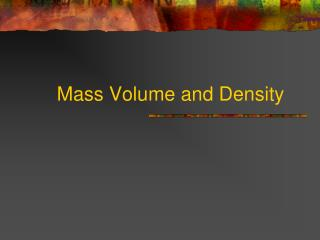DownloadDownload PresentationMass Volume and Density

# Mass Volume and Density

Télécharger la présentation## Mass Volume and Density

- - - - - - - - - - - - - - - - - - - - - - - - - - - E N D - - - - - - - - - - - - - - - - - - - - - - - - - - -
##### Presentation Transcript

1. Mass Volume and Density

2. What is matter? • Matter is what the universe is made of. • Matter is anything that has mass and volume. • Examples include just about everything (any solid, liquid or gas). • Example of things that are not matter include electricity, sound and light.

3. Mass- how much matter is present Measured in Kg, g and mg Volume- how much space something takes up Measured in mL, L and cm3 What is the difference between mass and volume? Which weighs more a ton of bricks or a ton of feathers? Neither, they both have the same mass. However, the feathers will have a much higher volume.

4. Weight is the force of gravity pulling down on the mass of an object. Weight = mass x gravity and 99.99999% of the time gravity is a constant (9.8 m/s2) A normal scale will divide for you and give you your mass However there are differences The difference between mass and weight.

5. Weight is a force, it pulls in a definite direction. Weight is measured in Newtons (N) Weight changes with altitude. On the moon you would weigh 1/6 of what you do on Earth. Mass is inertia (resistance to change in motion) it has no direction. Mass is measured in kilograms (Kg). Mass never changes, unless you remove or add matter to the object.

6. How to measure volume. If it is a cubic solid, measure length. width and height and multiply them. l x w x h = v If it is a liquid, pour it in a graduated cylinder and read from the meniscus (the low point of the curve). ALWAYS PUT YOUR HEAD AT THE SAME LEVEL AS THE MENISCUS air meniscus water

7. Measuring an irregular solid • first pour water into a graduated cylinder large enough to hold the solid, and read its volume. • then place the solid into the water being careful not to spill any water. • read the new volume. • subtract the new volume from the old for the volume of the solid.

8. How to measure mass • If it is a “nonmessy” solid, weigh it on a scale. • If it is a liquid or messy (a powder, greasy etc.) get a weighing tray or beaker. • weigh the empty weighing tray or beaker and record its mass. • add the object to the weighing tray or beaker and weigh it and subtract the mass of the empty beaker.

9. Scale Rules • Gently place objects on scale. • Make sure the scale is clean. (wipe off excess powder) • Make absolutely certain before you use it it reads 0.00 g • If it doesn’t press ON/ZERO

10. Density • Density is a ratio of mass to volume of an object. • D= m / v (density = mass / volume) • Density is measured in g/mL (grams per milliliter). • Density of a pure compound or element is a constant • Gold is always 19.3 g/mL, pure water is always 1.0 g/mL

11. Quick density test • less dense objects float in more dense objects. least dense most dense cork oil plastic water penny

12. Interesting point… • Density of water is 1 g/mL • The density of steel is 7.9 g/mL • What is the density of an aircraft carrier loaded with jets, crew, and guns? • If it hasn’t been sunk it is less than 1 g/mL • Boats have a hollow hull (big space for air to collect) to decrease their density. • Air has a density of .0013 g/mL